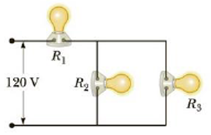Chapter 18, Problem 50AP

Chapter
Section
Textbook Problem

Three 60.0-W, 120-V lightbulbs are connected across a 120-V power source, as shown in Figure P18.50. Find (a) the total power delivered to the three bulbs and (b) the potential difference across each. Assume the resistance of each bulb is constant (even though, in reality, the resistance increases markedly with current).Figure P18.50

To determine
The value of each resistor.

Explanation

Formula to calculate the power delivered in series circuit is,

ΔPseries=I2(R1+R2) (I)

• I is the current.
• R1 and R2 are the resistances.

Formula to calculate the power delivered in parallel circuit is,

ΔPparallel=I2(R1R2R1+R2) (II)

Substitute 225 W for ΔPseries and 5 A for I in Equation (I).

225W=(5A)2(R1+R2)

On Re-arranging,

R1+R2=9Ω (III)

Substitute 50.0 W for ΔPparallel , 9Ω for R1+R2 and 5 A for I in Equation (II).

50

Still sussing out bartleby?

Check out a sample textbook solution.

See a sample solution

The Solution to Your Study Problems

Bartleby provides explanations to thousands of textbook problems written by our experts, many with advanced degrees!

Get Started

What are the features of a healthy high-fat diet?

Understanding Nutrition (MindTap Course List)

How is advection related to this system?

Fundamentals of Physical Geography

What is physically exchanged during crossing over?

Human Heredity: Principles and Issues (MindTap Course List)

How do neritic sediments differ from pelagic ones?

Oceanography: An Invitation To Marine Science, Loose-leaf Versin